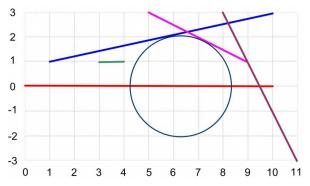# 2815. Largest Empty Circle on a Segment

We are given $N$ line segments on the 2D plane. We want to find the maximum radius of an empty circle whose center coordinates $(x_c, y_c)$ are constrained as follows:

• $0 \le x_c \le L$
• $y_c = 0$

A circle is empty if no part of a segment is located strictly inside of it (thus, a segment may touch the circle, but may not intersect with the interior of the circle).### 输入格式

The first line of the input file contains the number of test cases $T$. The test cases are described next. The first line of a test case contains the integer numbers $N$ and $L$ ($1 \le N \le 2000, 0 \le L \le 10000$). The next $N$ lines of the test case contain $4$ integers each, describing the coordinates of the endpoints of a segment: $x_a$, $y_a$, $x_b$ and $y_b$. The coordinates of the endpoints of the segment are $(x_a, y_a)$ and $(x_b, y_b)$. All the coordinates are between $-20000$ and $+20000$. Every two consecutive numbers on the same line are separated by a single blank.

### 输出格式

For each test case print a line containing a real number $R$, denoting the maximum radius of an empty circle whose center obeys the constraints. The number must be printed with $3$ decimal digits (the number must be rounded up or down according to the usual rounding rules).

### 样例

Input
1
4 10
1 1 10 3
5 3 9 1
3 1 4 1
8 3 11 -3

Output
2.118


1 人解决，1 人已尝试。

1 份提交通过，共有 2 份提交。

9.2 EMB 奖励。